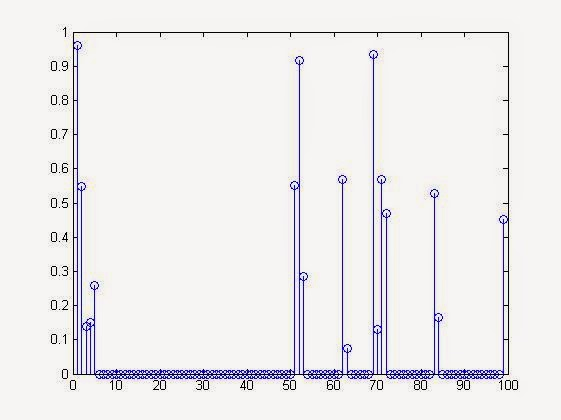# MATLAB Code to Generate a Block Sparse Signal Vector

So what so special about Block Sparse Signal?
These signal are sparse, i.e., having a lots of zero as its elements. But the non zero elements occurs in blocks of random length continuous index of a Sparse signal Vector.

Visualizing in a simple manner block sparse signal will look like,
0 0 0 0 0 0 0 5 3 2 0 0 0 0 0 0 4 2 0 0 0 0 0 0 1 1 3 4 0 0 0 0 0 0 0 0 0 0 0 6 5 3 0 0 0 0 0 0

Using the above concept I have written a code to generate the above signal. The code will give the user option to specify the total length of the sparse signal vector, maximum & minimum size of continuous zeros & non zero elements.

MATLAB Code to Generate a Block Sparse Signal

clc;
close all;
clear all;
n=100;
%total length of the sparse signal
zeromaxsize=10; %maximum size of continuous zeros
zerominsize=5; %minimum size of continuous zeros
maxblocksize=5; %maximum size of non-zero elements block
minblocksize=2; %minimum size of non-zero elements block
t=0;
xorg=[];
while t<n
x=rand;
%generate a value between 0 to1
v=0;
u=0;
if x<=0.5
%you can change this condition depending upon your condition
v=ceil(rand*zeromaxsize); %generating a continuous zero series
if v<zerominsize
xorg=[xorg zeros(zerominsize,1)'];
else
xorg=[xorg zeros(v,1)'];
end
else
u=ceil(rand*maxblocksize);
%to generate non zero element block
if u<minblocksize
xorg=[xorg rand(2,1)'];
else
xorg=[xorg rand(u,1)'];
end
v=ceil(rand*zeromaxsize);
%to generate immediate zeros after a non zero block
if v<zerominsize
xorg=[xorg zeros(zerominsize,1)'];
else
xorg=[xorg zeros(v,1)'];
end
end
t=t+v+u;
end
if length(xorg)>n
%this step to eradicate any extra element if the resultant signal vector length >100
l=length(xorg)-n;
xorg(end-l:end)=[];
end
spy(xorg)
%spy graph visualization of sparse signal
figure
stem(xorg)
%stem plot to visualize the same sparse signal

Sample Run of the above MATLAB Code & Output

Matlab Spy Graph Visualization of Block Sparse Signal thus generated.Matlab Spy Graph Visualization of Block Sparse Signal thus generated.
Matlab Stem Plot of Block Sparse Signal Thus GeneratedMatlab Stem Plot of Block Sparse Signal Thus Generated

Comment below for solving any doubt about above implementation.